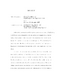## Phase transitions of high-temperature superconductors##### Files
umi-umd-4676.pdf(1.46 MB)
In this thesis phase transitions of the high temperature superconductor YBa$_2$Cu$_3$O$_{7-\delta}$ (YBCO) have been investigated in both zero and non-zero magnetic field. Current-Voltage characteristics of thin films and single crystals have been studied to determine the transition temperature and critical exponents. We optimized our film samples to ensure that they are of single phase, $c$-axis oriented and homogeneous. High-quality crystal samples were provided by Dr.~Kouji Segawa and Dr.~Yoichi Ando. In the zero-field transition, finite-size effects, which can obscure the phase transition by introducing ohmic tails below the transition temperature, are observed in the current-voltage curves of even the thickest film (2400 \AA) at low currents. The data at high currents are not affected by finite-size effects so that we can use derivative plots to determine $T_c$ and the dynamic critical exponent $z$. The current-voltage curves of crystals' data, however, are not affected by finite-size effects even in the lowest current measured as expected. $z$ determined from YBCO crystals are consistent with the one determined from YBCO films: $z=1.5 \pm 0.2$. This is a strong evidence that the dynamic universality class of high-temperature superconductors belongs to model-E dynamics in zero field. The static critical exponent $\nu$ determined from %the ohmic tails above $T_c$ is $0.85\pm 0.2$ for the melting line $(T_c-T_{g(m)})\sim H^{1/2\nu_0}$ is $0.68 \pm 0.1$ for crystal and $0.62\pm 0.1$ for thin films. The phase transitions in the mixed state (non-zero field) are more complicated. In the phase transition of YBCO thin films in field, finite-size effects are again observed. The presence of magnetic field leads to anisotropic vortex loops so that finite-size effects are enhanced. We observe a magnetic field $H$ dependence of the crossover current density $J_{min}$ as well as the exponent $z$. At $H>1$ T, $J_{min}$ and $z$ stay relatively constant. $z\simeq 2$ at high field implies a crossover from model-E dynamics to model-A dynamics. Finally, we will discuss $E-J$ characteristics of the first-order melting transition of untwinned YBCO single crystals.